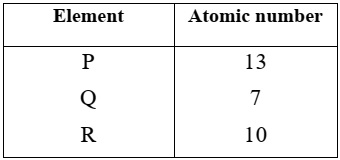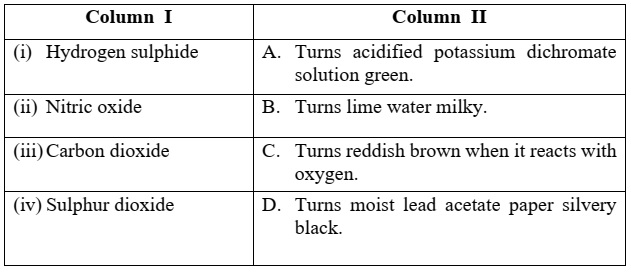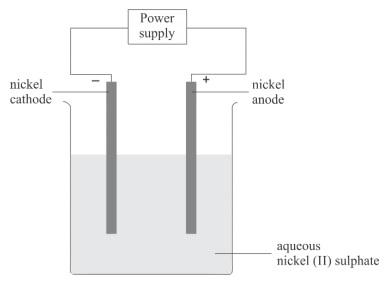# ICSE Class 10 Chemistry 2020 Question Paper

Chemistry is Science Paper - 2. The duration of the paper is two hours. Section I is compulsory. Attempt any four questions from Section II.

### Section I (40 Marks)

Question 1

(a) Choose the correct answer from the options given below: 

(i) The element with highest ionization potential, is:

1. Hydrogen
2. Caesium
4. Helium

(ii) The inert electrode used in the electrolysis of acidified water, is:

1. Nickel
2. Platinum
3. Copper
4. Silver

(iii) A compound with low boiling point, is:

1. Sodium chloride
2. Calcium chloride
3. Potassium chloride
4. Carbon tetrachloride

(iv) The acid which can produce carbon from cane sugar, is:

1. Concentrated Hydrochloric acid
2. Concentrated Nitric acid
3. Concentrated Sulphuric acid
4. Concentrated Acetic acid

(v) The organic compound having a triple carbon-carbon covalent bond, is:

1. C3H4
2. C3H6
3. C3H8
4. C4H10

(b) State one relevant observation for each of the following reactions: 

1. Action of concentrated nitric acid on copper.
2. Addition of excess ammonium hydroxide into copper sulphate solution.
3. A piece of sodium metal is put into ethanol at room temperature.
4. Zinc carbonate is heated strongly.
5. Sulphide ore is added to a tank containing oil and water, and then stirred or agitated with air.

(c) Write a balanced chemical equation for each of the following: 

1. Reaction of carbon powder and concentrated nitric acid.
2. Reaction of excess ammonia with chlorine.
3. Reaction of lead nitrate solution with ammonium hydroxide.
4. Producing ethane from bromo ethane using Zn / Cu couple in alcohol.
5. Complete combustion of ethane.

(d) (i) Draw the structural formula for each of the following: 

1. 2,2 dimethyl pentane
2. methanol
3. Iso propane

(ii) Write the IUPAC name for the following compounds: 

1. acetaldehyde
2. acetylene

(e) State one relevant reason for each of the following: 

1. Graphite anode is preferred to platinum in the electrolysis of molten lead bromide.
2. Soda lime is preferred to sodium hydroxide in the laboratory preparation of methane.
3. Hydrated copper sulphate crystals turn white on heating.
4. Concentrated nitric acid appears yellow, when it is left for a while in a glass bottle.
5. Hydrogen chloride gas fumes in moist air.

(f) Calculate: 

(i) The amount of each reactant required to produce 750 ml of carbon dioxide, when two volumes of carbon monoxide combine with one volume of oxygen to produce two volumes of carbon dioxide.

2CO + O2 → 2CO2

(ii) The volume occupied by 80 g of carbon dioxide at STP.

(iii) Calculate the number of molecules in 4.4 gm of CO2. [Atomic mass of C= 12, O=16]

(iv) State the law associated in question no. (f)(i) above.

(g) Give one word or a phrase for the following statements: 

1. The chemical bond formed by a shared pair of electrons, each bonding atom contributing one electron to the pair.
2. Electrode used as cathode in electrorefining of impure copper.
3. The substance prepared by adding other metals to a base metal in appropriate proportions to obtain certain desirable properties.
4. The tendency of an atom to attract electrons to itself when combined in a compound.
5. The reaction in which carboxylic acid reacts with alcohol in the presence of conc. H2SO4 to form a substance having a fruity smell.

(h) Fill in the blanks from the choices given in brackets: 

1. The polar covalent compound in gaseous state that does not conduct electricity is ______. (carbon tetra chloride, ammonia, methane)
2. A salt prepared by displacement reaction is ______. (ferric chloride, ferrous chloride, silver chloride)
3. The number of moles in 11gm of nitrogen gas is ______. (0.39, 0.49, 0.29) [atomic mass of N=14]
4. An alkali which completely dissociates into ions is ______. (ammonium hydroxide, calcium hydroxide, lithium hydroxide)
5. An alloy used to make statues is______. (bronze, brass, fuse metal)

### Section II (40 Marks)

Question 2

(a) The following table represent the elements and the atomic number. With reference to this, answer the following using only the alphabets given in the table.1. Which element combines with hydrogen to form a basic gas?
2. Which element has an electron affinity zero?
3. Name the element, which forms an ionic compound with chlorine.

(b) Draw the electron dot diagram for the compounds given below. Represent the electrons by (.) and (x) in the diagram. [Atomic No.: Ca = 20, O = 8, Cl = 17, H = 1] 

1. Calcium oxide
2. Chlorine molecule
3. Water molecule

(c) Choose the correct word which refers to the process of electrolysis from A to E, to match the description (i) to (iv): 

A: Oxidation B: Cathode C: Anode D: An electrolyte E: Reduction

1. Conducts electricity in aqueous or in molten state.
2. Loss of electron takes place at anode.
3. A reducing electrode.
4. Electrode connected to the positive end or terminal of the battery.

Question 3

(a) Baeyer’s process is used to concentrate bauxite ore to alumina. Give balanced chemical equations for the reaction taking place for its conversion from bauxite to alumina. 

(b) Complete the following by selecting the correct option from the choices given: 

1. pH of acetic acid is greater than dilute sulphuric acid. So acetic acid contains __________ concentration of H+ ions. (greater, same, low)
2. The indicator which does not change colour on passage of HCl gas is __________. (methyl orange, moist blue litmus, phenolphthalein)
3. The acid which cannot act as an oxidizing agent is __________. (conc. H2SO4, conc. HNO3, conc. HCl)

(c) Match the gases given in column I to the identification of the gases mentioned in column II:Question 4

(a) Differentiate between the following pairs based on the information given in the brackets. 

1. Conductor and electrolyte (conducting particles)
2. Cations and anions (formation from an atom)
3. Acid and Alkali (formation of type of ions)

(b) Draw the structures of isomers of pentane. 

(c) Hydrogen chloride gas is prepared in the laboratory using concentrated sulphuric acid and sodium chloride. Answer the questions that follow based on this reaction: 

1. Give the balanced chemical equation for the reaction with suitable condition(s) if any.
2. Why is concentrated sulphuric acid used instead of concentrated nitric acid?
3. How is the gas collected?
4. Name the drying agent not used for drying the gas.

Question 5

(a) Distinguish between the following pairs of compounds using a reagent as a chemical test: 

1. Calcium nitrate and Zinc nitrate solution.
2. Ammonium sulphate crystals and Sodium sulphate crystals.
3. Magnesium chloride and Magnesium nitrate solution.

(b) Calculate the percentage of: 

1. Fluorine
2. Sodium and
3. Aluminium

in sodium aluminium fluoride [Na3AlF6], to the nearest whole number. [Atomic Mass: Na = 23, Al = 27, F = 19]

(c) (i) State the volume occupied by 40 gm of methane at STP, if its vapour density (V.D.) is 8.

(ii) Calculate the number of moles present in 160 gm of NaOH. [Atomic Mass: Na = 23, H = 1, O = 16] 

Question 6

(a) Identify the salts P, Q, R from the following observations: 

1. Salt P has light bluish green colour. On heating, it produces a black coloured residue. Salt P produces brisk effervescence with dil. HCl and the gas evolved turns lime water milky, but no action with acidified potassium dichromate solution.
2. Salt Q is white in colour. On strong heating, it produces buff yellow residue and liberates reddish brown gas. Solution of salt Q produces chalky white insoluble precipitate with excess of ammonium hydroxide.
3. Salt R is black in colour. On reacting with concentrated HCl, it liberates a pungent greenish yellow gas which turns moist starch iodide paper blue black.

(b) Identify the substance underlined in each of the following: 

1. The electrode that increases in mass during the electro-refining of silver.
2. The acid that is a dehydrating as well as a drying agent.
3. The catalyst used to oxidize ammonia into nitric oxide.

(c) Copy and complete the following paragraph using the options given in brackets: 

Alkenes are a homologous series of (i) ______ (saturated / unsaturated) hydrocarbons characterized by the general formula (ii) ______ (CnH2n+2 / CnH2n). Alkenes undergo (iii) _____ (addition / substitution) reactions and also undergo (iv) ____ (hydrogenation / dehydrogenation) to form alkanes.

Question 7

(a) Write balanced chemical equations, for the preparation of the given salts (i) to (iii) by using the methods A to C respectively: 

A: Neutralization B: Precipitation C: Titration

1. Copper sulphate
2. Zinc carbonate
3. Ammonium sulphate

(b) Name the following elements: 

1. An alkaline earth metal present in group 2 and period 3.
2. A trivalent metal used to make light tools.
3. A monovalent non-metal present in fluorspar.

(c) An aqueous solution of nickel (II) sulphate was electrolyzed using nickel electrodes. Observe the diagram and answer the questions that follow:(i) What do you observe at the cathode and anode respectively?

(ii) Name the cation that remains as a spectator ion in the solution.

(iii) Which equation for the reaction at the anode is correct?

1. Ni → Ni2+ + 2e
2. Ni + 2e → Ni2+
3. Ni2+ → Ni + 2e
4. Ni2+ + 2e → Ni